Next: F.3 Gain Saturation Correction Up: Calibration Files for Standard Previous: F.1 Bin of Aluminum

# F.2 Correction for ADC Non-linearities (NLC)

The ADC produces small variations in the channel width. The effective width of each channel has been determined by adding up all available ground calibration data (up to 1990 March 17 about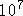events) into a single spectrum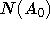and subtracting a smooth spline function. The correction table contains for each raw channel the lower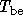and upper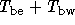bin edges, where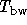is the bin width. The ADC non-linearity correction of the pulse height results then from equation F.5 and is plotted in Fig. F.2. To avoid discretization effects random numbers r are used to distribute an event amplitude within the bin. Apparently, on average a pulse height event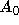is transformed into an event with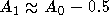, e.g.,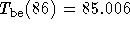and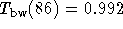. It seems unclear whether this feature is intentional. Also there is known a photon excess at lower energies [Hasinger and Snowden1992] which could have been produced by an offset of 3-5 eV (one channel is approximately 10eV).Figure F.2: Correction tables for ADC non-linearities (cf. Eq. (F.5)). Upper panel: the effective bin width in channels of each ADC\ pulse height bin (). It has been obtained by subtraction of a smooth spline function from an accumulated spectrum (events) of all available ground calibration data: width = 1+ residual[data-model]. Lower panel: difference of upper edge of ADC bin and smooth spline function. The information was taken from file p\$manpar:adc_bins.dat.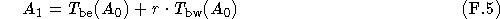Parameters:] ADC pulse height channel of event (integer in [1,256])lower bounds of the linearized ADC pulse height channels (cf. Fig. F.2 lower panel)widths of the linearized ADC pulse height channels (cf. Fig. F.2 upper panel)
r
random number (real number in [0.0,1.0])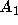pulse height of event, corrected for ADC non-linearity

SASS source code: package PSPC, bubble CT, subroutine CADC

SASS files:Next: F.3 Gain Saturation Correction Up: Calibration Files for Standard Previous: F.1 Bin of Aluminum

If you have problems/suggestions please send mail to rosat_svc@mpe-garching.mpg.de